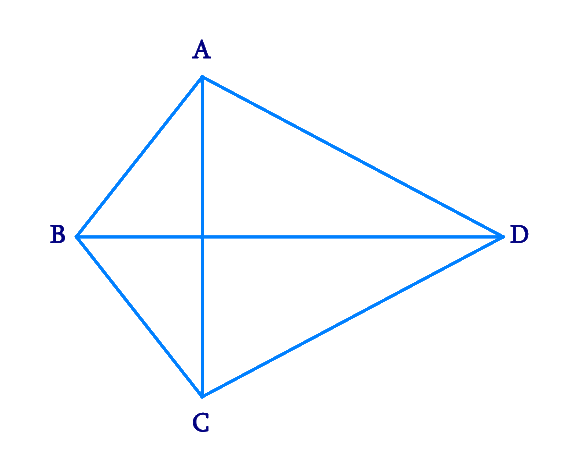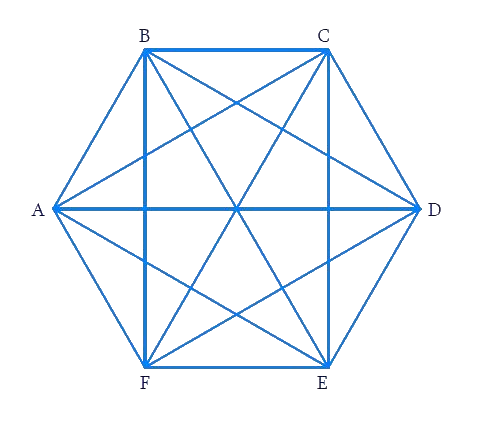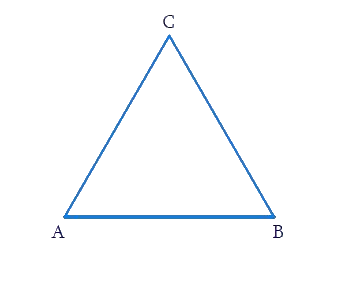# Ex.3.1 Q2 Understanding Quadrilaterals Solution - NCERT Maths Class 8

Go back to  'Ex.3.1'

## Question

How many diagonals does each of the following have?

(b) A regular hexagon

(c) A triangle

Video Solution
Ex 3.1 | Question 2

## Text Solution

What is known?

Polygons with known number of vertices.

What is unknown?

Number of diagonals

Reasoning:

A diagonal is a line segment connecting two non-consecutive vertices of a polygon. Draw the above given polygon and mark vertices and then, draw lines joining the two non-consecutive vertices. From this, we can calculate the number of diagonals.

A convex quadrilateral has two diagonals.Here, $$AC$$ and $$BD$$ are two diagonals.

(b) A regular hexagonHere, the diagonals are $$AD, \,AE,\, BD,\, BE,\, FC,\, FB, \,AC,\, EC$$ and $$FD.$$ Totally there are $$9$$ diagonals.

(c) A triangleA triangle has no diagonal because there no two non-consecutive vertices.

Learn from the best math teachers and top your exams

• Live one on one classroom and doubt clearing
• Practice worksheets in and after class for conceptual clarity
• Personalized curriculum to keep up with school